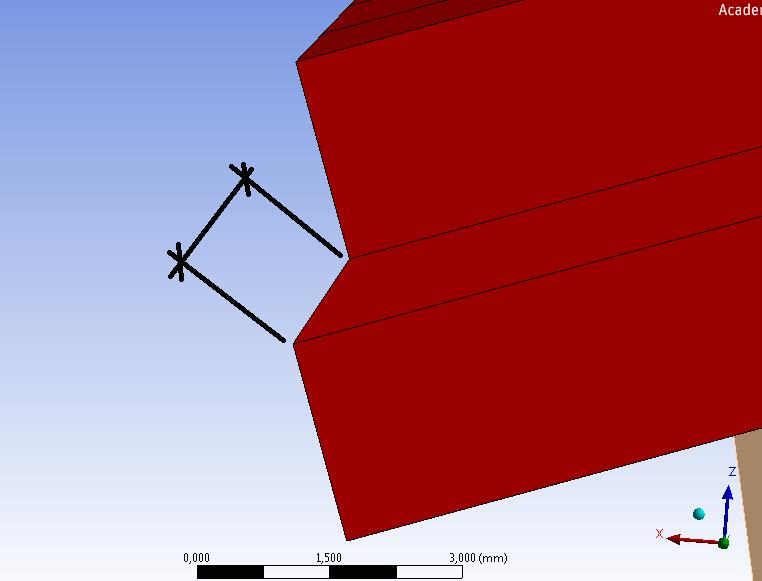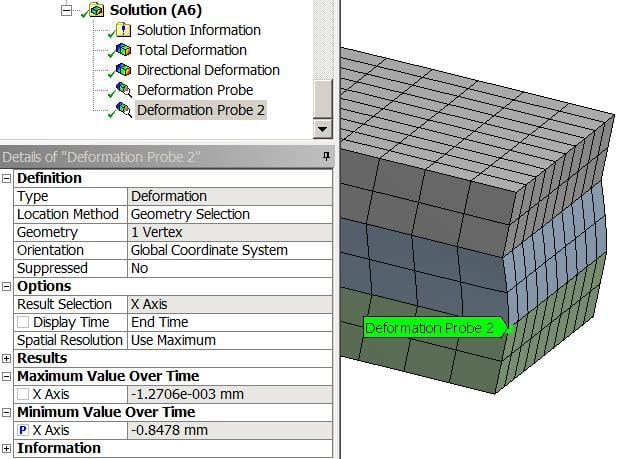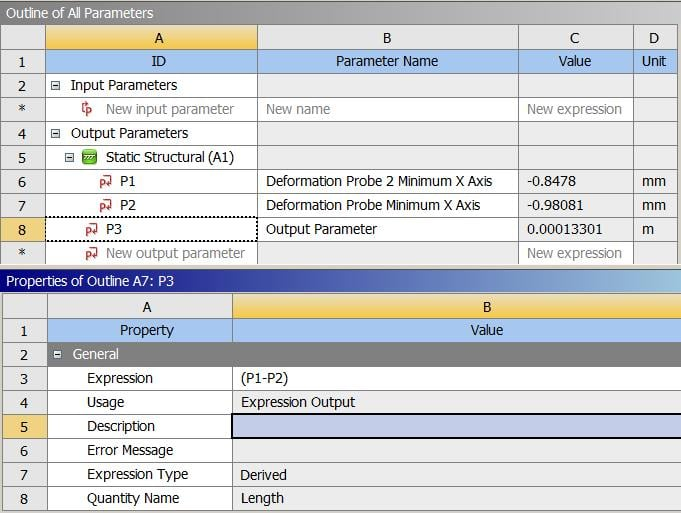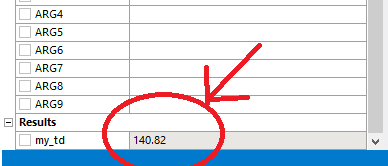## General Mechanical

•jonsys
Subscriber
•Rohith Patchigolla
Ansys Employee

Hello Jonsys,

You can do this via APDL command snippet under Solution.

Use the Selection Information icon in the toolbar (the i with an arrow after the Worksheet button) along with the node filter to get the node numbers for the two desired nodes. Then add a command object under solution of:

set,1

n1=arg1

n2=arg2

!

dx=(nx(n1)+ux(n1))-(nx(n2)+ux(n2))

dy=(ny(n1)+uy(n1))-(ny(n2)+uy(n2))

dz=(nz(n1)+uz(n1))-(nz(n2)+uz(n2))

my_td=sqrt(dx**2+dy**2+dz**2)

In the details window of the command object type one of the node numbers in the box for arg1 and the other node number in as arg2 (order is not important). Then solve the model and the result will be shown in the results window as my_td.

Let me know if this helps.

Best regards,

Rohith

•jonsys
Subscriber

Hello rgpatchi,

thank you. I get a value but can you please tell me what nx, ux and set,1 represent?

And why I can't get a result if I change from "my_td" to anything else?

Regards,

•Rohith Patchigolla
Ansys Employee

Hello Jonsys,

nx, ny, nz are functions which extract the x, y and z co-ordinates respectively of a node.

For example,

nx(201) --> gives x co-ordinate value of node 201.

similarly, ux, uy and uz are functions which extract x,y and z displacements of a node

ux(201) --> gives x displacement of node 201

So, using the above mentioned formula, I calculate the distance between the two nodes.

nx(201)+ux(201) --> deformed x co-ordinate value of node 201.

Regarding the result, if you use my_td, the command snippet (by default) extracts all the parameters with this prefix my_ into the details box of the command snippet.

Hence, if you change this to mytd instead, the result is still evaluated, but it is not automatically pulled into the details box of the command snippet. You would need to look into the solver output/post output to check the values.

You can change this search prefix in the details box of command snippet (Output Search Prefix) from my_ to anything else, or remove it altogether. But, it is advised to use a search prefix in order to not populate the command snippet details window with all the parameters defined in the command snippet.

Hope this clarifies.

Best regards,

Rohith

•jonsys
Subscriber

thank you rgpatchi,

if nx+ux gives the deformed coordinate, then I think my_td, based on the code, represents this distance (Fig below), right?So, is nx the displacement at Last time of the analysis? How can we extract displacement at a specific time?

my_td explanation is very clear, thank you.

Regards,

•Rohith Patchigolla
Ansys Employee

Hello Jonsys,

Yes you are right. I thought you wanted this distance.

Sorry for the confusion.

For this case, I think we can use a local co-ordinate system with say X normal to the edge and extract the difference between deformed x-coordinates of these two nodes in this local co-ordinate system.

Regarding extracting displacement at a specific time, you would need to use SET command before the ux and nx commands.

Example,

set,1,4 --> this reads the results for 4th substep of 1st load step from the results file.

Please go through the SET command documentation in help for further reference.

Best regards,

Rohith

•peteroznewman
Subscriber

Hello Jon,

I wish I was skilled in APDL like Rohith, but I'm not yet, so I figure out how to get the data I need without using code. In this case, I would put a probe on each vertex and click the parameters button.Deformation Probe is the vertex on the top of the flexible middle layer.

Then in the Parameter Set, you can write a little equation to subtract the two parameters.Note that the P3 value is in m not mm. I could multiply the expression by 1000 but it still lists the units as m.

Regards,

Peter

•jonsys
Subscriber

Hello rgpatchi,

Can you please tell me how can I define such a local coordinate system? I couldn't succeed in doing that so far.

Also, the distance depicted at my last comment is slightly smaller than the distance in the undeformed state, which is strange.
Moreover, in my model, I apply a displacement, therefore I did some simple trigonometrical calculation on where should this edge node be located at the end of the calculation~ I compare this with the following code: my_displacedcoord=nx(n1)+ux(n1). nx is the same but ux has a difference of 20mm. I want to read more in the documentation about the ux, where can I find it?

Regards,

•jonsys
Subscriber

Hello Peter,

From the method that you suggested, I think that the value of P1-P2 would get me the projection in x axis of the distance that I require (Please check the 2nd picture at the question and also the coordinate system). Is that right?

Regards,

•peteroznewman
Subscriber

Hello Jon,

Yes, the projection onto the X-axis, which for small rotations, will be close to the value along the deformed shape. However, you can turn on all three coordinate directions on the Probe and do a lot more math to get the exact value you want. I was just illustrating a way to do it without APDL code.

Regards,

Peter

•cnac
Subscriber
Thank you for this post, very HelpfulnHow do I increase the number of decimal places in the outputnrgpatchi HannoverMember Posts: 137 September 2018 edited September 2018nHello Jonsys, nYou can do this via APDL command snippet under Solution. nUse the Selection Information icon in the toolbar (the i with an arrow after the Worksheet button) along with the node filter to get the node numbers for the two desired nodes. Then add a command object under solution of:nset,1nn1=arg1nn2=arg2n!ndx=(nx(n1)+ux(n1))-(nx(n2)+ux(n2))ndy=(ny(n1)+uy(n1))-(ny(n2)+uy(n2))ndz=(nz(n1)+uz(n1))-(nz(n2)+uz(n2))nmy_td=sqrt(dx**2+dy**2+dz**2)nIn the details window of the command object type one of the node numbers in the box for arg1 and the other node number in as arg2 (order is not important). Then solve the model and the result will be shown in the results window as my_td.nLet me know if this helps.nBest regards,nRohithnry helpful.nn